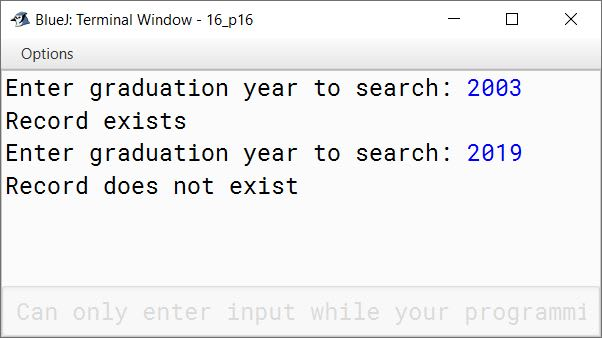# Solved 2014 Question Paper ICSE Class 10 Computer Applications

## Section A

#### Question 1

(a) Which of the following are valid comments ?

1. /*comment*/
2. /*comment
3. //comment
4. */comment*/

Option 1 — /*comment*/
Option 3 — //comment

(b) What is meant by a package ? Name any two java Application Programming Interface packages.

A package is a named collection of Java classes that are grouped on the basis of their functionality. Two java Application Programming Interface packages are:

1. java.util
2. java.io

(c) Name the primitive data type in Java that is:

1. a 64-bit integer and is used when you need a range of values wider than those provided by int.
2. a single 16-bit Unicode character whose default value is '\u0000'.

1. long
2. char

(d) State one difference between the floating point literals float and double.

float literalsdouble literals
float literals have a size of 32 bits so they can store a fractional number with around 6-7 total digits of precision.double literals have a size of 64 bits so they can store a fractional number with 15-16 total digits of precision.

(e) Find the errors in the given program segment and re-write the statements correctly to assign values to an integer array.

```int a = new int (5);
for (int i=0; i<=5; i++) a[i]=i;```

Corrected Code:

```int a[] = new int;
for (int i = 0; i < 5; i++)
a[i] = i;```

#### Question 2

(a) Operators with higher precedence are evaluated before operators with relatively lower precedence. Arrange the operators given below in order of higher precedence to lower precedence:

1. &&
2. %
3. >=
4. ++

++
%
>=
&&

(b) Identify the statements listed below as assignment, increment, method invocation or object creation statements.

1. System.out.println("Java");
2. costPrice = 457.50;
3. Car hybrid = new Car();
4. petrolPrice++;

1. Method Invocation
2. Assignment
3. Object Creation
4. Increment

(c) Give two differences between the switch statement and the if-else statement

switchif-else
switch can only test if the expression is equal to any of its case constantsif-else can test for any boolean expression like less than, greater than, equal to, not equal to, etc.
It is a multiple branching flow of control statementIt is a bi-directional flow of control statement

(d) What is an infinite loop? Write an infinite loop statement.

A loop which continues iterating indefinitely and never stops is termed as infinite loop. Below is an example of infinite loop:

```for (;;)
System.out.println("Infinite Loop");```

(e) What is constructor? When is it invoked?

A constructor is a member method that is written with the same name as the class name and is used to initialize the data members or instance variables. A constructor does not have a return type. It is invoked at the time of creating any object of the class.

#### Question 3

(a) List the variables from those given below that are composite data types:

1. static int x;
2. arr[i]=10;
3. obj.display();
4. boolean b;
5. private char chr;
6. String str;

The composite data types are:

• arr[i]=10;
• obj.display();
• String str;

(b) State the output of the following program segment:

```String str1 = "great"; String str2 = "minds";
System.out.println(str1.substring(0,2).concat(str2.substring(1)));
System.out.println(("WH" + (str1.substring(2).toUpperCase())));```

The output of the above code is:

``````grinds
WHEAT
``````

Explanation:

1. str1.substring(0,2) returns "gr". str2.substring(1) returns "inds". Due to concat both are joined together to give the output as "grinds".
2. str1.substring(2) returns "eat". It is converted to uppercase and joined to "WH" to give the output as "WHEAT".

(c) What are the final values stored in variable x and y below?

```double a = -6.35;
double b = 14.74;
double x = Math.abs(Math.ceil(a));
double y = Math.rint(Math.max(a,b)); ```

The final value stored in variable x is 6.0 and y is 15.0.

Explanation:

1. Math.ceil(a) gives -6.0 and Math.abs(-6.0) is 6.0.
2. Math.max(a,b) gives 14.74 and Math.rint(14.74) gives 15.0.

(d) Rewrite the following program segment using if-else statements instead of the ternary operator:

String grade = (marks>=90)?"A": (marks>=80)? "B": "C";

```String grade;
if (marks >= 90)
else if (marks >= 80)
else

(e) Give the output of the following method:

```public static void main (String [] args){
int a = 5;
a++;
System.out.println(a);
a -= (a--) - (--a);
System.out.println(a);} ```

##### Output
``````6
4
``````
##### Explanation
1. a++ increments value of a by 1 so a becomes 6.
2. a -= (a--) - (--a)
⇒ a = a - ((a--) - (--a))
⇒ a = 6 - (6 - 4)
⇒ a = 6 - 2
⇒ a = 4

(f) What is the data type returned by the library functions :

1. compareTo()
2. equals()

1. int
2. boolean

(g) State the value of characteristic and mantissa when the following code is executed:

```String s = "4.3756";
int n = s.indexOf('.');
int characteristic=Integer.parseInt(s.substring(0,n));
int mantissa=Integer.valueOf(s.substring(n+1));```

The value of characteristic is 4 and mantissa is 3756.

Index of . in String s is 1 so variable n is initialized to 1. s.substring(0,n) gives 4. Integer.parseInt() converts string "4" into integer 4 and this 4 is assigned to the variable characteristic.

s.substring(n+1) gives 3756 i.e. the string starting at index 2 till the end of s. Integer.valueOf() converts string "3756" into integer 3756 and this value is assigned to the variable mantissa.

(h) Study the method and answer the given questions.

```public void sampleMethod()
{   for (int i=0; i < 3; i++)
{   for (int j = 0; j<2; j++)
{int number = (int)(Math.random() * 10);
System.out.println(number); }}}```
1. How many times does the loop execute?
2. What is the range of possible values stored in the variable number?

1. The loops execute 6 times.
2. The range is from 0 to 9.

(i) Consider the following class:

```public class myClass {
public static int x=3, y=4;
public int a=2, b=3;}```
1. Name the variables for which each object of the class will have its own distinct copy.
2. Name the variables that are common to all objects of the class.

1. a, b
2. x, y

(j) What will be the output when the following code segments are executed?

(i)

```String s = "1001";
int x = Integer.valueOf(s);
double y = Double.valueOf(s);
System.out.println("x="+x);
System.out.println("y="+y);```

(ii)

`System.out.println("The king said \"Begin at the beginning!\" to me.");`

(i) The output of the code is:

``````x=1001
y=1001.0
``````

(ii) The output of the code is:

``````The king said "Begin at the beginning!" to me.
``````

## Section B

#### Question 4

Define a class named movieMagic with the following description:

Data MembersPurpose
int yearTo store the year of release of a movie
String titleTo store the title of the movie
float ratingTo store the popularity rating of the movie
(minimum rating=0.0 and maximum rating=5.0)
Member MethodsPurpose
movieMagic()Default constructor to initialize numeric data members to 0 and String data member to "".
void accept()To input and store year, title and rating
void display()To display the title of the movie and a message based on the rating as per the table given below
##### Ratings Table
RatingMessage to be displayed
0.0 to 2.0Flop
2.1 to 3.4Semi-Hit
3.5 to 4.4Hit
4.5 to 5.0Super-Hit

Write a main method to create an object of the class and call the above member methods.

```import java.util.Scanner;

public class movieMagic
{
private int year;
private String title;
private float rating;

public movieMagic() {
year = 0;
title = "";
rating = 0.0f;
}

public void accept() {
Scanner in = new Scanner(System.in);
System.out.print("Enter Title of Movie: ");
title = in.nextLine();
System.out.print("Enter Year of Movie: ");
year = in.nextInt();
System.out.print("Enter Rating of Movie: ");
rating = in.nextFloat();
}

public void display() {
String message = "Invalid Rating";
if (rating <= 2.0f)
message = "Flop";
else if (rating <= 3.4f)
message = "Semi-Hit";
else if (rating <= 4.4f)
message = "Hit";
else if (rating <= 5.0f)
message = "Super-Hit";

System.out.println(title);
System.out.println(message);
}

public static void main(String args[]) {
movieMagic obj = new movieMagic();
obj.accept();
obj.display();
}
}```
##### Output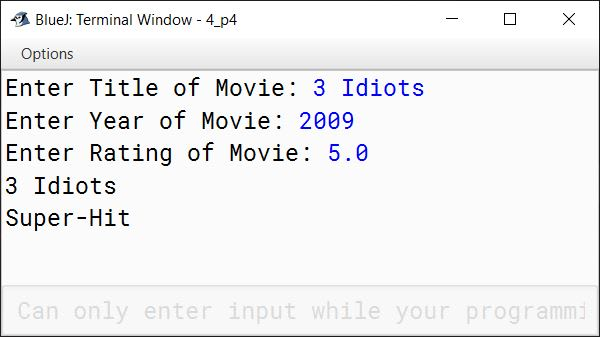#### Question 5

A special two-digit number is such that when the sum of its digits is added to the product of its digits, the result is equal to the original two-digit number.

Example: Consider the number 59.
Sum of digits = 5 + 9 = 14
Product of digits = 5 * 9 = 45
Sum of the sum of digits and product of digits = 14 + 45 = 59

Write a program to accept a two-digit number. Add the sum of its digits to the product of its digits. If the value is equal to the number input, then display the message "Special two—digit number" otherwise, display the message "Not a special two-digit number".

```import java.util.Scanner;

public class KboatSpecialNumber
{
public void checkNumber() {

Scanner in = new Scanner(System.in);

System.out.print("Enter a 2 digit number: ");
int orgNum = in.nextInt();

int num = orgNum;
int count = 0, digitSum = 0, digitProduct = 1;

while (num != 0) {
int digit = num % 10;
num /= 10;
digitSum += digit;
digitProduct *= digit;
count++;
}

if (count != 2)
System.out.println("Invalid input, please enter a 2-digit number");
else if ((digitSum + digitProduct) == orgNum)
System.out.println("Special 2-digit number");
else
System.out.println("Not a special 2-digit number");

}
}```
##### Output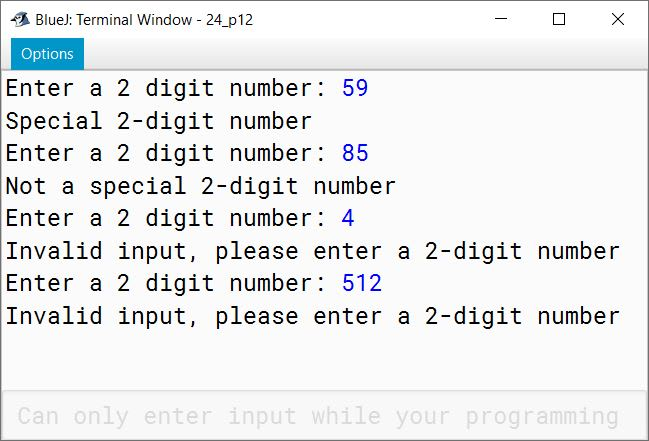#### Question 6

Write a program to assign a full path and file name as given below. Using library functions, extract and output the file path, file name and file extension separately as shown.

Input

Output
File name: flower
Extension: jpg

```import java.util.Scanner;

public class KboatFilepathSplit
{
public static void main(String args[]) {

Scanner in = new Scanner(System.in);
System.out.print("Enter full path: ");
String filepath = in.next();

char pathSep = '\\';
char dotSep = '.';

int pathSepIdx = filepath.lastIndexOf(pathSep);
System.out.println("Path:\t\t" + filepath.substring(0, pathSepIdx));

int dotIdx = filepath.lastIndexOf(dotSep);
System.out.println("File Name:\t" + filepath.substring(pathSepIdx + 1, dotIdx));

System.out.println("Extension:\t" + filepath.substring(dotIdx + 1));
}
}```
##### Output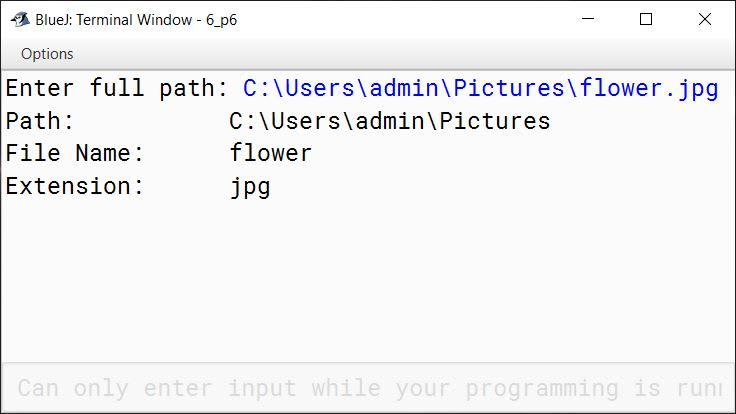#### Question 7

Design a class to overload a function area( ) as follows:

1. double area (double a, double b, double c) with three double arguments, returns the area of a scalene triangle using the formula:
area = √(s(s-a)(s-b)(s-c))
where s = (a+b+c) / 2
2. double area (int a, int b, int height) with three integer arguments, returns the area of a trapezium using the formula:
area = (1/2)height(a + b)
3. double area (double diagonal1, double diagonal2) with two double arguments, returns the area of a rhombus using the formula:
area = 1/2(diagonal1 x diagonal2)

```import java.util.Scanner;

{
double area(double a, double b, double c) {
double s = (a + b + c) / 2;
double x = s * (s-a) * (s-b) * (s-c);
double result = Math.sqrt(x);
return result;
}

double area (int a, int b, int height) {
double result = (1.0 / 2.0) * height * (a + b);
return result;
}

double area (double diagonal1, double diagonal2) {
double result = 1.0 / 2.0 * diagonal1 * diagonal2;
return result;
}
}```

#### Question 8

Using the switch statement, write a menu driven program to calculate the maturity amount of a Bank Deposit.
The user is given the following options:

1. Term Deposit
2. Recurring Deposit

For option 1, accept principal (P), rate of interest(r) and time period in years(n). Calculate and output the maturity amount(A) receivable using the formula:

A = P[1 + r / 100]n

For option 2, accept Monthly Installment (P), rate of interest (r) and time period in months (n). Calculate and output the maturity amount (A) receivable using the formula:

A = P x n + P x (n(n+1) / 2) x r / 100 x 1 / 12

For an incorrect option, an appropriate error message should be displayed.

```import java.util.Scanner;

public class KboatBankDeposit
{
public static void main(String args[]) {
Scanner in = new Scanner(System.in);
System.out.println("Type 1 for Term Deposit");
System.out.println("Type 2 for Recurring Deposit");
int ch = in.nextInt();
double p = 0.0, r = 0.0, a = 0.0;
int n = 0;

switch (ch) {
case 1:
System.out.print("Enter Principal: ");
p = in.nextDouble();
System.out.print("Enter Interest Rate: ");
r = in.nextDouble();
System.out.print("Enter time in years: ");
n = in.nextInt();
a = p * Math.pow(1 + r / 100, n);
System.out.println("Maturity amount = " + a);
break;

case 2:
System.out.print("Enter Monthly Installment: ");
p = in.nextDouble();
System.out.print("Enter Interest Rate: ");
r = in.nextDouble();
System.out.print("Enter time in months: ");
n = in.nextInt();
a = p * n + p * ((n * (n + 1)) / 2) * (r / 100) * (1 / 12.0);
System.out.println("Maturity amount = " + a);
break;

default:
System.out.println("Invalid choice");
}
}
}```
##### Output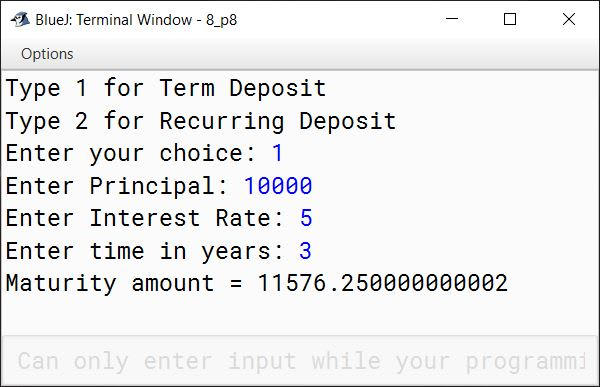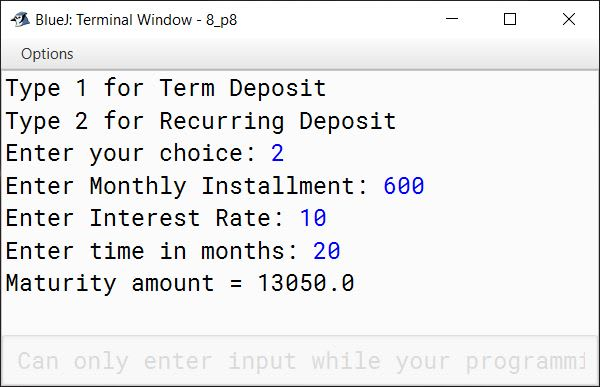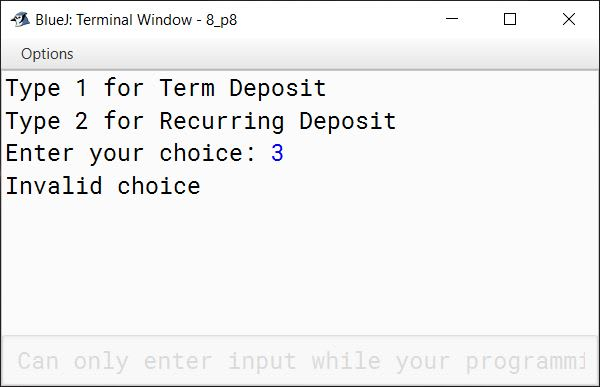#### Question 9

Write a program to accept the year of graduation from school as an integer value from the user. Using the binary search technique on the sorted array of integers given below, output the message "Record exists" if the value input is located in the array. If not, output the message "Record does not exist".
Sample Input:

nnnnnnnnnn
1982198719931996199920032006200720092010

```import java.util.Scanner;

{
public static void main(String args[]) {
Scanner in = new Scanner(System.in);
int n[] = {1982, 1987, 1993, 1996, 1999, 2003, 2006, 2007, 2009, 2010};

System.out.print("Enter graduation year to search: ");
int year = in.nextInt();

int l = 0, h = n.length - 1, idx = -1;
while (l <= h) {
int m = (l + h) / 2;
if (n[m] == year) {
idx = m;
break;
}
else if (n[m] < year) {
l = m + 1;
}
else {
h = m - 1;
}
}

if (idx == -1)
System.out.println("Record does not exist");
else
System.out.println("Record exists");
}
}```
##### Output